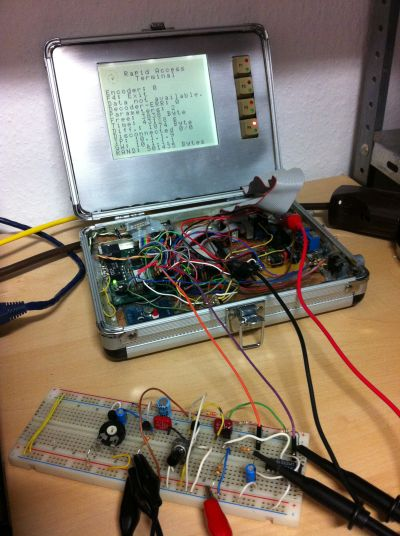## Random Number Server

RNS (random number servers) generate true random numbers unsing at high data rates using physical random processes

IP 88.217.181.242 4224 (default) 50 kBit/s Transistor diode pn-junction thermal noise amlifier with close loop controlled probability justifictaion. Unbiased output using Von Neumann method. offline### Rating

```> rnsget 10.1.1.9 -b -n 100000 | ent
Entropy = 7.988830 bits per byte.

Optimum compression would reduce the size
of this 100430 byte file by 0 percent.

Chi square distribution for 100430 samples is 1646.94, and randomly
would exceed this value less than 0.01 percent of the times.

Arithmetic mean value of data bytes is 125.2881 (127.5 = random).
Monte Carlo value for Pi is 3.209463496 (error 2.16 percent).
Serial correlation coefficient is 0.010609 (totally uncorrelated = 0.0).```

### Tools

Free tools tools to access the server are:
• The command line tool rnsget to download random numbers fom a RNS-Server
• Driver for Linux to map the random number server to DevRandom /dev/random provideing good quality random numbers for all linux applications
• RandomNumberBufferServer RNBS is a random number proxy for Linux to provide random numbers for local networks
• rnsOctave is a warper for the command rand() for Octave
• rnsMatlab is a warper for the command rand() for Matlab

Siehe auch